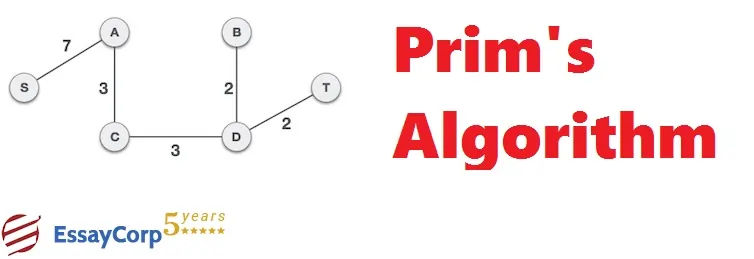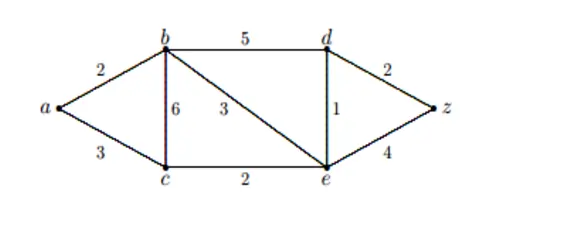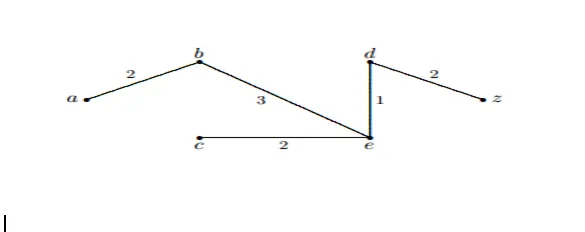# What is Prim’s Algorithm? An Explanation with ExamplesComputer science is a vast field. Prim’s algorithm time complexity is a significant part of it. A greedy algorithm that enables finding the spanning tree for the weighted undirected graph. It implies solving the wedges subset boosts a tree formation and accompanies every vertex, where it minimizes the overall weight of the edge in the tree.

The Algorithm’s conduction indicates having the tree constructed means the tree being built at a singular vertex at a time, from the arbitrary starting vertex, at every step, adding the cheapest connection from the tree to a different vertex.

Then comes Prim's algorithm time complexity; the Algorithm is O((V+E)log V) as only once an edge insertion happens in the priority queue. Experts use this Algorithm to find the tree with n nodes and edges. The sum of the weights of these edges is the minimum of all possible trees.

## Is Prim's Algorithm greedy?

Yes, prim’s Algorithm is explained as a greedy algorithm as it reorders the inputs. It has an adaptive nature. It can reiterate itself for making the Algorithm convenient. Prim's algorithm visualization typically involves showing the process of adding edges to the tree individually. Each edge is chosen based on its weight and the vertices it connects.

For instance, a typical visualization of Prim’s algorithm time complexity might start with a graph with five vertices and ten edges and show the Algorithm gradually adding edges to form the minimum spanning tree.

The visualization might start with all vertices edges uncolored and gradually color them as experts add them to the tree. The final result would be a fully colored graph showing the minimum spanning tree obtained by the Algorithm.

Below, we shall explain an example of the weighted connected graph G having n vertices. Prim’s Algorithm enables finding the minimum spanning tree of the weighted connected graph G. Here is Prim's algorithm example with a solution -

Technique:

Prim: (G denotes the weighted connected graph having n number of vertices) T denotes the minimum-weight edge wherein i = 1 up till n − 2 begin e denotes a minimum weight edge which is incident in T, to a vertex and does not form a circuit in T if added to T T:= T with e added

end

return(T)

E.g., Utilize the Algorithm of Prim to find a solution of the minimum spanning tree in the below given weighted graph. To break ties, enable using the alphabetical order.Solution:

The Algorithm of Prim shall progress as below:

• Most preliminarily, add the edge {d, e} having a 1 weight.

• Then, enable adding edge {d, e} of 2 weight

• Next, enable adding {d, z} having weight 2

• Then, enable adding edge {b, e} of 3 weight

• Finally, add edge {a, b} with a weight of 2.

It shall thus generate a weight 10 minimum spanning tree, which is given below:## What is the prim's algorithm complexity?

Prim’s algorithm complexity is O(E+ V log V) using a Fibonacci heap, where E is the number of edges in the graph, and V is the number of vertices.

The space complexity of Prim’s Algorithm is O(V), as it requires a boolean array of size V to keep track of which vertices add up to the minimum spanning tree and a priority queue of size E or V, depending on whether there is a use of binary heap Fibonacci heap.

Note that the performance of Prim’s Algorithm Python can vary depending on the implementation details and the process characteristics of the graph. In practice, the Fibonacci heap implementation may be faster for graphs with many vertices and edges, while the binary heap implementation may be faster for smaller charts.

## Prim's Algorithm vs Kruskal - A Brief Comparison

Prim’s Algorithm C++ and Kruskal’s Algorithm are popular algorithms for finding the minimum spanning tree of a given weighted graph, but they differ in their approach and time complexity.

Prim’s Algorithm is explained as a greedy algorithm that starts with a single vertex and adds edges to form a tree until all vertices include and obtain a minimum spanning tree. As explained above, Prim’s Algorithm is a binary or Fibonacci heap.

On the other hand, Kruskal's Algorithm is also a greedy algorithm that starts with a forest of trees and gradually merges them to form a single minimum-spanning tree. The Algorithm maintains a set of disjoint sets of vertices and selects the next edge to add based on the minimum weight of all trims that connect two locations. The time complexity of Kruskal’s Algorithm is O(E log E) using a sorting algorithm such as quicksort.

In terms of performance, Prim’s Algorithm Python can be faster than Kruskal’s Algorithm for dense graphs, where the number of edges is close to the maximum possible (V*(V-1)/2), as the O(E log V) or O(E + V log V) time complexity is better than the O (E log E) time complexity of Kruskal’s Algorithm.

However, in sparse graphs, where the number of edges is much smaller than the maximum possible, Kruskal’s Algorithm may be faster, as the O (E Log E) time complexity is better than the O (E log V) or E ( E + v log v) time complexity of Prim’s Algorithm.

To sum up, all the above points are useful in comparing Prim's Algorithm vs Kruskal's. Professionals who use both algorithms understand their properties.

## Best Assignment Writing Help for the Technical Subjects

Scholars studying technical subjects such as Prim's Algorithm C++ devote much time to practicing such complex algorithms. They spend hours in their classes and in front of computers to develop new algorithms and software.

On top of that, completing lengthy assignments is a task in itself. Consequently, scholars seek assignment writing help online. At EssayCorp, we have distinguished experts from their respective fields who help students complete their assignments on time.

Whether a scholar is looking for Prim’s Algorithm Python or wants to draft an assignment based on Prim’s algorithm calculator. Our team of academic writers consists of experts with professional degrees in the same domain.

Our academic writers provide fully referenced assignments with proper citations. Our projects are faultless and plagiarism-free. Moreover, our experts offer 24/7 assistance to students. They can send their queries on our WhatsApp at any point in time.